# Two Phase Simplex Method: Linear Programming

Let's solve the following problem with the two phase simplex method. We will use the same process as used in the last example.

##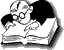Two Phase Simplex Method Example

Maximize z = 12x1 + 15x2 + 9x3

subject to

8x1 + 16x2 + 12x3 ≤ 250
4x1 + 8x2 + 10x3 ≥ 80
7x1 + 9x2 + 8x3 = 105

x1, x2, x3 ≥ 0

Solution.

Introducing slack, surplus & artificial variables

8x1 + 16x2 + 12x3 + x4 = 250
4x1 + 8x2 + 10x3 – x5 + A1 = 80
7x1 + 9x2 + 8x3 + A2 = 105

Where:
x4 is a slack variable.
x5 is a surplus variable.
A1& A2 are artificial variables.

#### Phase 1

Maximize 0x1 + 0x2 + 0x3 + 0x4 + 0x5 + (–A1) + (–A2)

subject to

8x1 + 16x2 + 12x3 + x4 = 250
4x1 + 8x2 + 10x3 – x5 + A1 = 80
7x1 + 9x2 + 8x3 + A2 = 105

x1, x2, x3, x4, x5, A1, A2 ≥ 0

Equating x1, x2, x3, x5 to zero.

Initial basic feasible solution

x4 = 250, A1= 80 , A2 = 105

Table 1

On small screens, use horizontal scrollbar to view full calculation

cj 0 0 0 0 0 -1 -1
cB Basic variables
B
x1 x2 x3 x4 x5 A1 A2 Solution values
b (= XB)
0 x4 8 16 12 1 0 0 0 250
-1 A1 4 8 10 0 -1 1 0 80
-1 A2 7 9 8 0 0 0 1 105
zj–cj   -11 -17 -18 0 1 0 0

Table 2

cj 0 0 0 0 0 -1
cB Basic variables
B
x1 x2 x3 x4 x5 A2 Solution values
b (= XB)
0 x4 16/5 32/5 0 1 6/5 0 154
0 x3 2/5 4/5 1 0 -1/10 0 8
-1 A2 19/5 13/5 0 0 4/5 1 41
zj-cj   -19/5 -13/5 0 0 -4/5 0

Here, Phase 1 terminates because both the artificial variables have been removed from the basis.

#### Phase 2 of Two Phase Simplex Method

Table 3

cj 12 15 9 0 0
cB Basic variables
B
x1 x2 x3 x4 x5 Solution values
b (= XB)
0 x4 0 80/19 0 1 10/19 2270/19
9 x3 0 10/19 1 0 -7/38 70/19
12 x1 1 13/19 0 0 4/19 205/19
zj-cj   0 -39/19 0 0 33/38

Table 4

Use horizontal scrollbar to view full calculation

cj 12 15 9 0 0
cB Basic variables
B
x1 x2 x3 x4 x5 Solution values
b (= XB)
0 x4 0 0 -8 1 2 90
15 x2 0 1 19/10 0 -7/20 7
12 x1 1 0 -13/10 0 9/20 6
zj-cj   0 0 39/10 0 3/20

The optimal solution is:
x1 = 6, x2 = 7, x3 = 0
z = 12 X 6 + 15 X 7 + 9 X 0 = 177.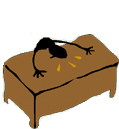Well, after going through this section you definitely deservesome food. Before moving to the next section, you must take a break because you really deserve it.

"Studying all the while without relaxation degrades a person's performance." -Vinay Chhabra & Manish Dewan

•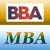MBA & BBA
•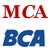BCA & MCA
•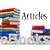Ebooks & Articles
•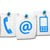Contact us